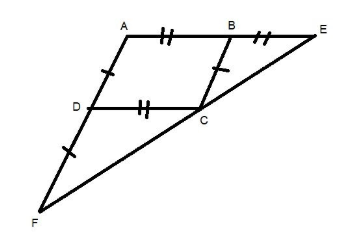QUESTION

# ${\text{ABCD}}$ is a parallelogram. The sides ${\text{AB}}$and ${\text{AD}}$are produced to ${\text{E}}$ and ${\text{F}}$respectively, such that ${\text{AB = BE}}$ and ${\text{AD = DF}}$.Hence, $\Delta {\text{BEC}} \cong \Delta {\text{DCF}}$If the above statement is true then mention the answer as $1$,else mention $0$ if false.

Hint:-In this question you first need to make relations between different angles, sides and given condition to get the relation between the triangles $\Delta {\text{BEC and }}\Delta {\text{DCF}}$and then checking the condition that these are congruent or not, using SAS(side-Angle-Side), SSS(side-side-side), ASA(Angle-Side-Angle) properties.Given: ABCD is a parallelogram.
We know,
AD=BC ($\therefore$ABCD is a parallelogram)
And AD=DF ($\therefore$Given)
Thus,
DF=BC eq.1
Similarly
AB=CD ($\therefore$ABCD is a parallelogram)
And AB=BE ($\therefore$Given)
Thus,
BE=CD eq.2
Since, corresponding angles for parallel lines of a parallelogram are equal
Then,

$\Rightarrow \angle {\text{BAD = }}\angle {\text{CDF (}}\therefore {\text{corresponding angles for parallel lines AB and CD)}} \\ \Rightarrow \angle {\text{BAD = }}\angle {\text{CBE (}}\therefore {\text{corresponding angles for parallel lines AD and BC)}} \\$

Thus,
$\angle {\text{CDF = }}\angle {\text{CBE eq}}{\text{.3}}$
Now, consider $\Delta {\text{DCF and }}\Delta {\text{BEC}}$
DF=BC {$\therefore$from eq.1}
$\angle {\text{CDF = }}\angle {\text{CBE}}$ {$\therefore$from eq.3}
CD=BE {$\therefore$from eq.2}
Thus, ${\text{ }}\Delta {\text{DCF }} \cong {\text{ }}\Delta {\text{BEC}}$ {$\therefore$Side-Angle-Side Rule}
Since, the given statement is true then the answer is 1.

Note:-Whenever you get this type of problem the key concept of solving is to make a rough diagram of the situation which is given . Then using properties of given shape (as in this question parallelogram) and SSS,SAS, ASA etc. form relations between different angles and sides to get the required result.